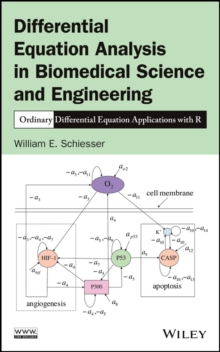Supporting your high street Find out how »
• My Account# Differential Equation Analysis in Biomedical Science and Engineering : Ordinary Differential Equation Applications with R Hardback

#### Description

Features a solid foundation of mathematical and computational tools to formulate and solve real-world ODE problems across various fields With a step-by-step approach to solving ordinary differential equations (ODEs), Differential Equation Analysis in Biomedical Science and Engineering: Ordinary Differential Equation Applications with R successfully applies computational techniques for solving real-world ODE problems that are found in a variety of fields, including chemistry, physics, biology, and physiology.

The book provides readers with the necessary knowledge to reproduce and extend the computed numerical solutions and is a valuable resource for dealing with a broad class of linear and nonlinear ordinary differential equations.

The author s primary focus is on models expressed as systems of ODEs, which generally result by neglecting spatial effects so that the ODE dependent variables are uniform in space.

Therefore, time is the independent variable in most applications of ODE systems.

As such, the book emphasizes details of the numerical algorithms and how the solutions were computed. Featuring computer-based mathematical models for solving real-world problems in the biological and biomedical sciences and engineering, the book also includes: * R routines to facilitate the immediate use of computation for solving differential equation problems without having to first learn the basic concepts of numerical analysis and programming for ODEs * Models as systems of ODEs with explanations of the associated chemistry, physics, biology, and physiology as well as the algebraic equations used to calculate intermediate variables * Numerical solutions of the presented model equations with a discussion of the important features of the solutions * Aspects of general ODE computation through various biomolecular science and engineering applications Differential Equation Analysis in Biomedical Science and Engineering: Ordinary Differential Equation Applications with R is an excellent reference for researchers, scientists, clinicians, medical researchers, engineers, statisticians, epidemiologists, and pharmacokineticists who are interested in both clinical applications and interpretation of experimental data with mathematical models in order to efficiently solve the associated differential equations. The book is also useful as a textbook for graduate-level courses in mathematics, biomedical science and engineering, biology, biophysics, biochemistry, medicine, and engineering.

#### Information

• Format: Hardback
• Pages: 440 pages
• Publisher: John Wiley & Sons Inc
• Publication Date:
• Category: Biomedical engineering
• ISBN: 9781118705483

£93.50

£78.49

on all orders

###### Pick up orders

from local bookshops

£138.00

£131.10

£109.99

£89.50

£76.08

£59.95